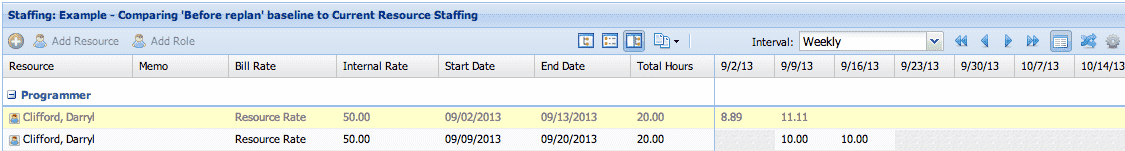# Example: Automatic Contours based on Hours

The following example uses this project calendar; working hours Monday through Friday from 9am to 5pm, with the following holidays:Allocation Unit: Hours
Contour Interval: any

When you create an allocation, the value you enter in Total Hours is spread evenly over the days in the date range. Even though "daily" is not an interval, the system divides the total value into a daily unit. The working hours are controlled by the project calendar. The weekly/monthly values are computed by the number of days in each interval.

For example, create a new allocation for 20 hours.You see that 20 working hours are evenly spread over working days. Because September 2 is a holiday, no hours were allocated on that day. Week 1 has a total of 8.89 hours (4 x 2.2222) and Week 2 has a total of 11.11 hours (5 x 2.2222).

You can view the same allocation in Headcount units:The converted Headcount value of 0.28 is shown for every week (automatic). However, because every week can have different working time (due to a holiday, for example), the total hours per week can differ. This is all calculated automatically by PPM Pro.

## Shifting

### Shift by week

Now let's shift the allocation by one week:The new date range is displayed, and those 20 hours are automatically re-spread over the new working days. The new date range has no holidays, and the first week has the same number of working days as the second week, resulting in even contours.

### Shift by days

The 20 hours are re-contoured over the date range. Shifting by one week is the same as shifting by 7 days.

### Shift by months

Let's create a resource allocation from August 1-September 30 for 200 hours, and then shift by 2 months. We'll also create a baseline for comparison:The system recognizes Sept 30 as the last day of the month, and therefore shifts to the last day of October. The 20 hours are now spread over the new date range.

Now let's view the allocation in Headcount.Notice that the new Headcount hours are different - here's why: the first date range (Aug 1 - Sept 30) had 42 working days, which computes to 336 hours. The second date range (Oct 1 - Nov 30) has 41 working days, which is 328 hours.

When we divide 200 hours by the total working hours, we get 2 different numbers: 200/336 = .5952 (rounds to .60), and 200/328 = .6098 (rounds to .61).

## Extending

### Extend by days

Let's extend by 10 days:Because the new date range has more working time (number of days), the Total Hours has increased.

Now let's view it in Headcount:Remember that the Headcount value is not stored - the allocation was defined in Hours. When extending, the Total Hours is first converted to Headcount, and then uniformly applied over the date range.

### Extend by Weeks

Works the same as extending by days.

### Extend by Months

Works the same as extending by days - except recognizes the last day of the month. This may cause a day to be added or subtracted (or several days if working with February).